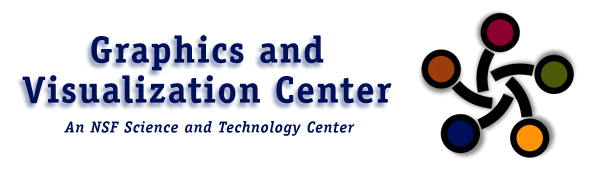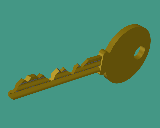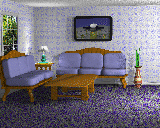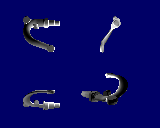# Generative Modeling# Generative Modeling and Related Methods

Generative modeling is a method of creating and manipulating three-dimensional geometric models. It has been implemented in a powerful, robust software package which has been used in a great variety of applications involving geometric shapes and their mathematical representations.

## Generative Modeling

Like many types of modeling systems, generative models are models described by mathematical functions and operators arranged in a hierarchy. What distinguishes it is the set of operators that are available.

Generative modeling is founded on the idea that a wide variety of mathematical operators, including constraint solution, differentiation, integration and more traditional modeling operators of hierarchical transformations and constructive solid geometry, form a natural language for shape specification. To this end, generative modeling offers a mathematically closed set of operators made up of the standard hierarchical operations for rigid objects (push, pop, rotate, translate, name object, etc.), constructive solid geometry operations (intersection, union, subtraction), and operators for creating time-dependent surfaces and other shapes (such as sweep operators, differentiation, integration, inverse functions, constraint solution, constrained minimization).

These operators are all made robust using the powerful mathematical technique of interval analysis, and provide a rich architecture and environment in which to base a modeling system. Details of this work have been published in a 1992 SIGGRAPH paper and John Snyder's 1992 Book .

## Applications of Generative Modeling

The Generative Modeling approach provides a general tool for other systems to interact mathematically with the modelled shapes. Through this interface, generative models can be used as the geometrical specification for a large variety of applications.

### Rendering

Any object specified by the generative modeling system can be rendered in a number of ways. It is possible to generate polygonal representations of the surface at arbitrary sampling resolution for use in depth-buffer systems, such as the ones implemented in graphics hardware on most modern graphics workstations. The high-level representation, however, can also be rendered with more sophisticated methods, such as direct raytracing. Because all generative modeling objects are defined as differentiable manifolds, it is possible to find exact intersections of rays with the surface, and then to calculate the normal at the exact intersection point. This representation can also be used in manufacturing to directly calculate the trajectory of a cutting tool.

### Simulation

The usefulness of this mathematical representation extends to simulation and analysis in addition to the visual domain. Generative models have been used in a structured modeling system as the basic specification of shape. This system is designed to be used as a universal mathematical and computational environment for physical simulations and physcially-based animations. The generative modeling system is used to provide the mathematical representation of shape used to calculate physical properties, effects, and conditions. The shapes described by a generative model can be integrated to get the moment of intertia tensor, and can be used directly to calculate collisions in a phsical rigid- or flexible-body simulation. Functions, such as stress, strain, or temperature, can be defined as a function of body position, and the generative modeling system can mesh objects by surface or volume for finite element simulations.

Because of design philosophy of mathematical closure under the generative modeling operators, it is possible to create parameterized models in which the designer can have direct control over the specific degrees of freedom which are applicable. It is also possible to define objects which vary over time (e.g. objects which have time as a geometric parameter), to create objects imbedded in other spaces (such as density functions on volumes or vector fields on surfaces), or to specify objects as the solutions to specific mathematical equations, such as differential, integral, or constrained optimization equations.

## The Newgen System

These methods have been implemented in a software system known as Newgen, which is a modeling environment based on the GENMOD language described in John Snyder's 1992 Book Generative Modeling for Computer Graphics and CAD.

Newgen uses and interpreted extension of the C language called cinterp as its environment for specifying the shape functions, and includes a curve editor for specifying curves by interactive design. It can also create models from implicit functions and extract them from volume datasets, such as MRI images.

An important element of the design philosophy of newgen is the idea that the user should be able to see the shape in full detail during the design stages. Therefore, newgen provides a viewing system which allows interactive examination in a continuous, real-time polygonal representation with interactively-controlled sampling resolution and rendering properties.

Newgen has been used as the geometric, and often the mathematical, interface for several raytracers and physical simulators, and to produce the short animated movies Fruit Tracing (1993) and Going Bananas (1987). Newgen is available for HP9000 series 700 workstations via FTP .